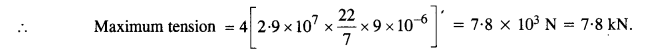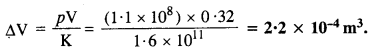## NCERT Solutions for Class 11 Physics Chapter 9 Mechanical Properties of Solids

These Solutions are part of NCERT Solutions for Class 11 Physics. Here we have given NCERT Solutions for Class 11 Physics Chapter 9 Mechanical Properties of Solids

Question 1.
A steel wire of length 4.7 m and cross-section 3.0 x 10-5 m2 stretches by the same amount as a copper wire of length 3.5 m and cross-section 4.0 x 10-5 m2 under a given load. What is the ratio of Young’s modulus of steel to that of copper?
For steel, length of wire, Ls = 4.7 m
Area of cross-section, As = 3 x 10-5 m2
For copper, length of wire, Lc = 3.5 m
Area of cross-section, Ac = 4.0 x 10-5 m2
Also, extension
ls = lc = lQuestion 2.
Fig. shows the stress-strain curve for a given material. What are
(a) Young’s modulus and
(b) approximate yield strength for this material?(a) From graph, for stress = 150 x 106 Nm-2
the corresponding strain = 0.002Question 3.
The stress-strain graphs for materials A and B are shown in Figure.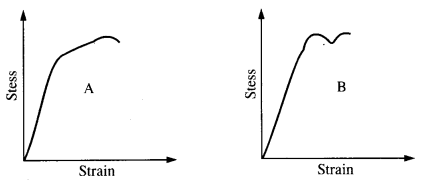The graphs are drawn to the same scale.
(a) Which of the material has greater Young’s modulus?
(b) Which material is more ductile?
(c) Which is more brittle?
(d) Which of the two is stronger material?
(a) material A has a greater Young’s modulus because the slope of the graph of material A is more.
(b) Material A is more ductile than material B because it has a large plastic deformation between the elastic limit and the breaking point.
(c) Material B is more brittle than material A because it has a small plastic deformation between the elastic limit and the breaking point.
(d) Material A is stronger than B because more stress is required to break it.

Question 4.
Read each of the statements below carefully and state, with reasons, if it is true or false.
(a) The modulus of elasticity of rubber is greater than that of steel.
(b) The stretching of a coil is determined by its shear modulus.
(a) False. Young’s modulus for steel is more than rubber.
(b) True

Question 5.
Calculate the elongation of the steel and brass wire in the Fig. Unloaded length of steel wire is 1.5 m and of brass wire = 1.0.m, the diameter of each wire = 0.25 cm. Young’s modulus of steel is 2.0 x 1011 Pa and that of brass is 0.91 x 1011 Pa.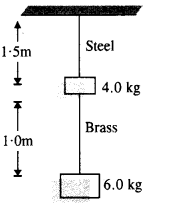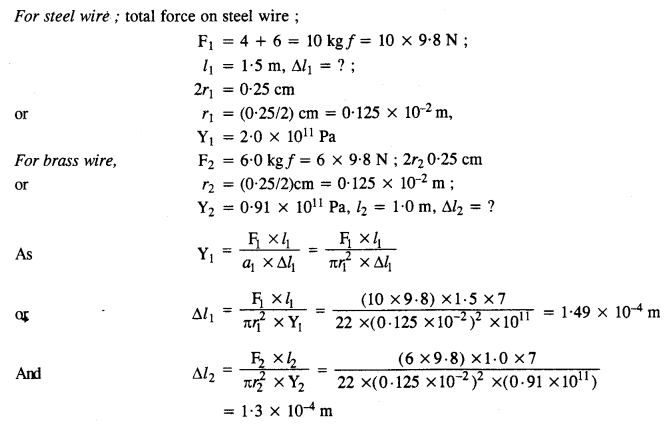Question 6.
The edges of an aluminium cube are 10 cm long. One face of the cube is firmly fixed to a vertical wall. A mass of 100 kg is then attached to the opposite face of the cube. The shear modulus of aluminium is 25 GPa. What is the vertical deflection of this face? (1 Pa = 1 Nm-2)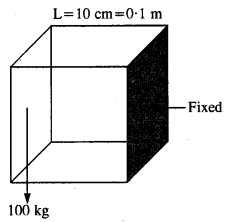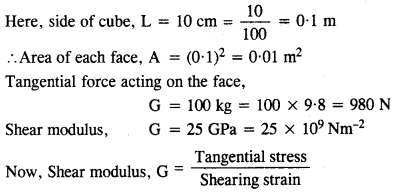Question 7.
Four identical hollow cylindrical columns of steel support a big structure of mass 50,000 kg. The inner and outer radii of each column are 30 and 60 cm respectively. Assuming the load distribution to be uniform, calculate the compressional strain of each column. The Young’s modulus of steel is 2.0 x 1011 Pa (1 Pa = 1 N m-2).
Here, outer radius of each column, r0 = 60 cm = 60 x 10“2 m
Inner radius, r,= 30 cm = 30 x 10-2 m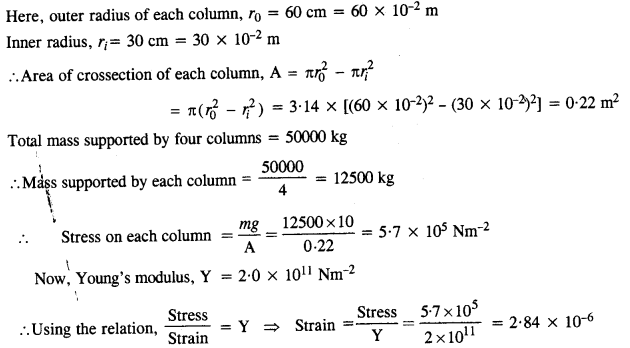Question 8.
A piece of copper having a rectangular cross-section of 15.2 mm x 19.1 mm is pulled in tension with 44,500 N force-producing only elastic deformation. Calculate the resulting strain. The shear modulus of elasticity of copper is 42 x 109 Nm-2.
Here, A = 15.2 x 19.1 x 10-6m2 . F = 44,500 N ; G = 42 X 109 Nm-2.Question 9.
A steel cable with a radius of 1.5 cm supports a chairlift at a ski area. If the maximum stress is not to exceed 108 Nm-2, what is the maximum load the cable cab support?
r = radius of cable = 1.5 cm.
A = π(0.015)2 m2
max stress = 108 Nm-2
Let F be the maximum force.
stress = $$\frac{F}{A}$$
∴ F = max stress × area.
= 108 × π × (0.015)2
= 7.065 × 104N
= 70,650 N
∴ The maximum load is 70,650 N

Question 10.
A rigid bar of mass 15 kg is supported symmetrically by three wires each 2 m long. These at each end are of copper and middle one is of iron. Determine the ratio of their diameters if each is to have the same tension. Young’s modulus of elasticity for copper and steel are 120 x 109 Nm-2 and 190 x 109 Nm-2 respectively.
As each wire has same tension F, so each wire has same extension due to mass of rigid bar. As each wire is of same length, hence each wire has same strain. If D is the diameter of wire, thenQuestion 11.

A 14.5 kg mass, fastened to the end of a steel wire of unstretched length 1 m, is Whirled in a vertical circle with an angular velocity of 2 rad/s at the bottom of the circle. The cross-sectional area of the wire is 0.065 cm2. Calculate the elongation of the wire when the mass is at the lowest point of its path.
Ysteel = 2 x 1011 Nm-2.
Here, m = 14.5 kg ;l = r = lm;v = 2rps;A = 0065 x 10-4 m2
Total pulling force on mass, when it is at the lowest position of the vertical circle is
F = mg + mr ω2
= 14 .5 x 9.8 + 14.5 x 1 x 4
= 200.1 N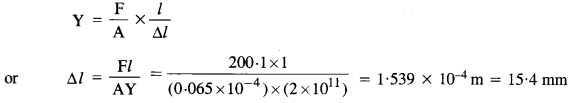Question 12.
Compute the bulk modulus of water from the following data : Initial volume = 100.0 litre, Pressure Increase =100.0 atm (1 atm = 1.013 x 105Pa), Final volume = 100.5 liter. Compare the bulk modulus of water with that of air (at constant temperature). Explain in simple terms why the ratio is so large.
(1 Pa = 1 Nm-2 ).
Given, V = 100 litre; Vf = 100.5 litre
:. Change in volume, ΔV = Vf – Vi= 100.5 – 100 = 0.5 liter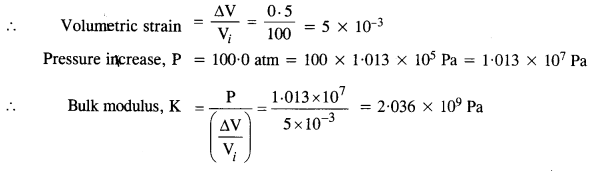Question 13.
What is the density of water at a depth where pressure is 80.0 atm, given that its density at the surface is 1.03 x 103 kg m-3? (Compressibility of water is 45.8 x 10-11 Pa-1 ; 1 Pa = 1 N m-2)
The increase in density is given by dρ = CPρ
where C is compressibility, P is pressure and p is the density at the surface.
Here, C = 45.8 x 10-11 Pa-1, P = 80 x 1 x 105 Nm-2, p = 1.03 x 103kg m3
∴   dρ= 45.8 x 10-11 x 80 x 105 x 1.03 x 103 = 3.774 kg m3
∴Total density at the given depth = ρ+ dp = 1.03 x 103 + 3.774
= 1.03 x 1000 + 3.774 = 1033.774 kg m-3 ≈1 .034 X 103 kg m-3

Question 14.
Compute the fractional change in volume of a glass slab, when subjected to a hydraulic pressure of 10 atmospheres. Bulk modulus of elasticity of glass = 37 x 109 Nm-2 and 1 atm = 1.103 x 10s Pa
Here ρ= 10 atm = 10 x 1.013 x 105 Pa ; K = 37 x 109 Nm-2Question 15.
Determine the volume contraction of a solid copper cube, 10 cm on an edge when subjected to a hydraulic pressure of 7 x 106 Pa. Bulk modulus of copper = 140 G Pal
Here,  L = 10 cm = 0.10 m ; p = 7 x 106 Pa ;
K = 140 G Pa = 140 x 109 Pa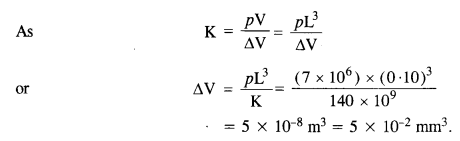Question 16.
How much should be pressure on a liter of water be changed to compress it by 0.10% ? Bulk modulus of elasticity of water = 2.2 x 109 Nm-2.
V = 1 liter = 10-3 m3 ; ΔV/V = 0.10/100 = 10-3Question 17.
Anvils made of single crystals of diamond, with the shape as shown in Fig. are used to investigate behavior of materials under very high pressures. Flat faces at the narrow end of the anvil have a diameter of 0.5 mm, and the wide ends are subjected to a compressional force of 50,000 N. What is the pressure at the tip of the anvil?Here, compressional force, F = 50000 N
Diameter, D = 0.5 mm = 0.5 x 10-3 m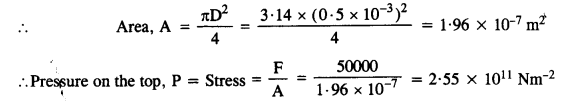Question 18.
A rod of length 1.05 m having negligible mass is supported at its ends by two wires of steel (wire A) and aluminium (wire B) of equal lengths as shown in Fig. The cross-sectional area of wires A and B are 1 mm2 and 2 mm2 respectively. At what point along the rod should a mass m be suspended in order to produce equal stresses and equal strains in both steel and aluminium wires? Given,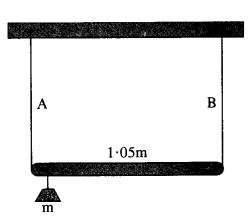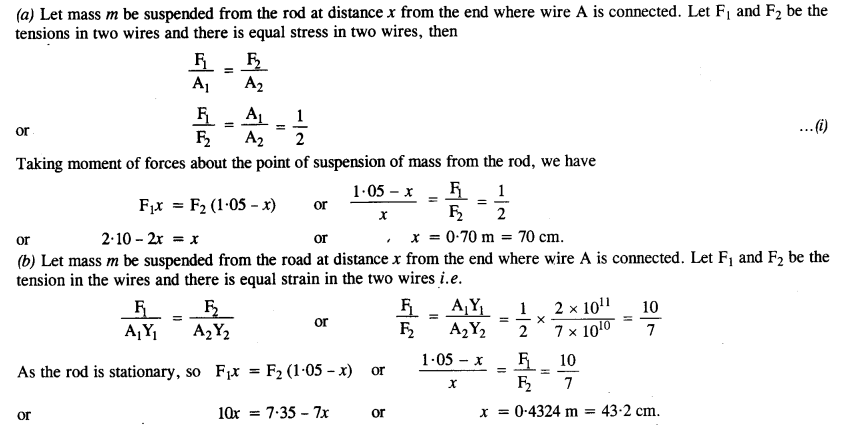Question 19.
A mild steel wire of length 1 m and cross-sectional area 0.5 x 10-2 cm2 is stretched, well within its elastic limit, horizontally between two pillars. A mass of 100 g is suspended from the midpoint of the wire. Calculate the depression at the midpoint, g = 10 ms-2 ; Y = 2 x 1011 Nm-2.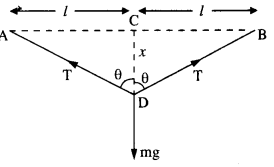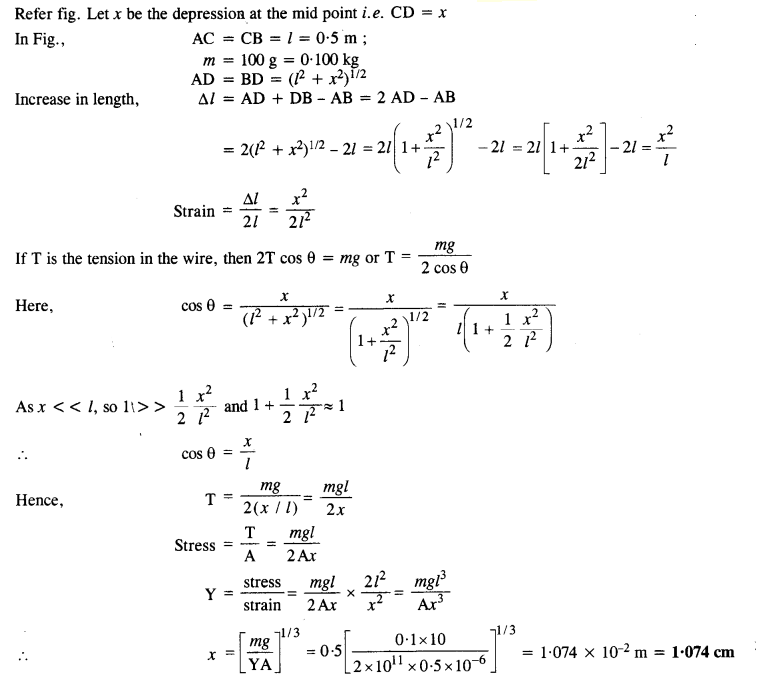Question 20.
Two strips of metal are riveted together at their ends by four rivets, each of diameter 6 mm. What is the maximum tension that can be exerted by the riveted strip if the shearing stress on the rivet is not to